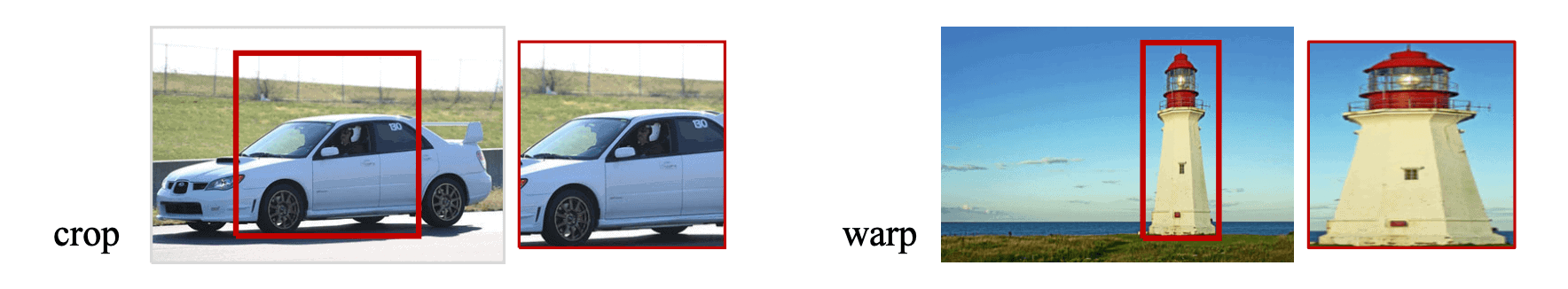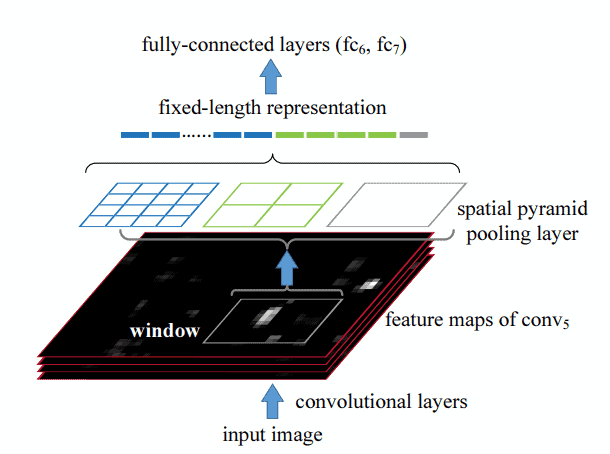## 1. Overview

In this tutorial, we’ll talk about the Spatial Pyramid Pooling (SPP) layer. First, we’ll make a brief introduction to CNNs for Visual Recognition. Then, we’ll present the problem of fixed size in the input image of a CNN. Finally, we’ll discuss the Spatial Pyramid Pooling layer that solves this problem.

## 2. CNNs for Visual Recognition

Convolutional Neural Networks (CNNs) have revolutionalized the field of computer vision and deep learning by achieving excellent performance in a variety of visual tasks. Due to their ability to learn discriminative features and generalize well to unseen new data, CNN architectures have surpassed previous classical ML architectures in many tasks.

One field that benefited most from this neural network architecture is visual object recognition, where the goal is to identify the objects that are present in an input image. Specifically, object recognition consists of two visual tasks, object localization, and image classification. In a previous article, we presented the task of object recognition more extensively, along with the metrics that are often used.

## 3. The Problem of Input Size

Despite their large success, CNNs present a very important technical issue that limits some of their capabilities. We refer to the fact that the input image of a CNN should have a fixed size. In order to give an image of arbitrary size as input to a CNN, we should resize the image either by cropping or warping. In the image below, we can see an example of these two resizing techniques:We can easily observe some problems with resizing the input image. Specifically:

• When we crop the input image, we run the risk of removing visual information that is useful for the recognition task. For example, in the above image, the front and the back part of the car are missing after cropping. These parts of the car are important in recognition.
• When we warp the image, we change the geometry of the objects in the image resulting in unwanted distortion.

As a result of the previous problems, there is a decrease in the performance of CNN models when they take input images with variable input sizes.

## 4. Spatial Pyramid Pooling

So, we realize that it is very crucial to find a way for CNNs to handle inputs with variable input sizes. First, let’s take a step back and think: Why do CNN architectures require inputs with fixed input sizes?

The answer lies in the fully-connected layers. Specifically, we know from previous articles that a CNN consists of some Convolutional (Conv) layers followed by some Fully-Connected (FC) layers. Conv layers don’t require fixed-size input since they operate using a sliding window. So, the FC layers set this limitation to CNNs.

The solution to this problem lies in the Spatial Pyramid Pooling (SPP) layer. It is placed between the last Conv layer and the first FC layer and removes the fixed-size constraint of the network.

The goal of the SPP layer is to pool the variable-size features that come from the Conv layer and generate fixed-length outputs that will then be fed to the first FC layer of the network. Below, we can see how the SPP layer works:The input of the SPP layer is a set offeature maps of arbitrary size. Then, the SPP layer maintains spatial information of these feature maps in local spatial bins. The output size of the SPP layer is fixed because the number and the size of these bins are always fixed. Specifically, we have three kinds of bins that act as pooling layers:

1. The first pooling layer (right in the image) consists of a single bin that outputs a-dimensional feature since it applies max pooling in each input feature map.
2. The second pooling layer (middle in the image) consists of 4 bins that output a-dimensional feature.
3. The third pooling layer (left in the image) consists of 16 bins that output a-dimensional feature

All pooling layers apply max pooling. In total, the output feature has a fixed size that is equal to.

## 5. Conclusion

In this article, we presented the Spatial Pyramid Pooling (SPP) layer. First, we introduced the CNN architecture and its limitation of fixed input size. Then, we described the SPP layer that solves this problem without reducing the overall performance of the model.